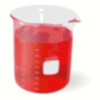#### You may also like### Plane to See

P is the midpoint of an edge of a cube and Q divides another edge in the ratio 1 to 4. Find the ratio of the volumes of the two pieces of the cube cut by a plane through PQ and a vertex.### 2D-3D

Two circles of equal size intersect and the centre of each circle is on the circumference of the other. What is the area of the intersection? Now imagine that the diagram represents two spheres of equal volume with the centre of each sphere on the surface of the other. What is the volume of intersection?### Brimful 2

Which of these infinitely deep vessels will eventually full up?

# Reach for Polydron

##### Age 16 to 18 Challenge Level:

To discover the easy way to find this volume you can very quickly make for yourself a model of the tetrahedron. Take a square $ABCD$ of stiff paper and fold it along the diagonal $AC$ . Open it until the distance between $B$ and $D$ is equal to the length of the sides of the square and then the four vertices are the vertices of the tetrahedron with two equilateral faces and two isosceles faces. You can either stand this 'tetrahedron' on an equilateral face ( $ABD$ or $CBD$ ) as its base or on an isosceles face ( $ABC$ or $ADC$ ) as its base. One choice makes it easy to find the area of the base and the height and so to find the volume.